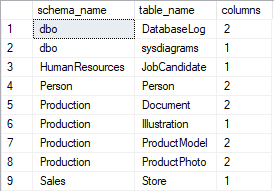## Find tables with large object (LOB) data type columns in SQL Server database

Article for:

Large objects in SQL Server are columns with following data types: varchar(max), nvarchar(max), text, ntext, image, varbinary(max), and xml.

Query below lists all tables that cointain columns with LOB data types.

## Query

``````select t.table_schema as schema_name,
t.table_name,
count(*) as columns
from information_schema.columns c
inner join INFORMATION_SCHEMA.tables t
on c.TABLE_SCHEMA = t.TABLE_SCHEMA
and c.TABLE_NAME = t.TABLE_NAME
where t.TABLE_TYPE = 'BASE TABLE'
and ((c.data_type in ('VARCHAR', 'NVARCHAR') and c.character_maximum_length = -1)
or data_type in ('TEXT', 'NTEXT', 'IMAGE', 'VARBINARY', 'XML', 'FILESTREAM'))
group by t.table_schema,
t.table_name
order by t.table_schema,
t.table_name
``````

## Columns

• schema_name - schema name
• table_name - table name
• columns - number of LOB columns in a table

## Rows

• One row represents one table
• Scope of rows: all tables containing columns with LOB data types in current database
• Ordered by schema name, table name

## Sample results

List of tables with LOB columns in AdventureWorks database: